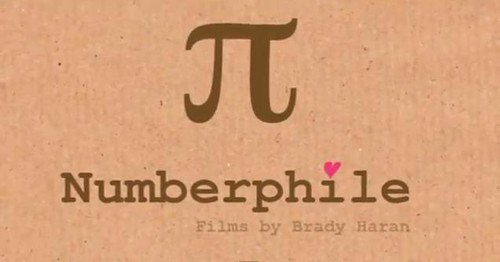# The ‘perfect chaos’ of π (The Guardian)

One of the most important numbers is irrational

GRRLSCIENTIST, by The Guardianπ has fascinated mathematicians, engineers and other people for centuries. It is a mathematical constant that is the ratio of a circle’s circumference (C) to its diameter (d);This also explains why and how this number got its name: the lowercase Greek letter π was first adopted in 1706 as an abbreviation for this number because it is the first letter of the Greek for “perimeter”, specifically of a circle. This symbol is convenient because π is an irrational number, meaning that it cannot be expressed as a ratio of a/b, where a and b are integers, that its digits never terminate, and it does not contain an infinitely repeating sequence.

Even though we know that the decimal for π is approximately 3.14159, we actually do not know all its digits precisely: as of October 2011, we know that π has more than 10 trillion non-repeating digits, and the occurrence of these digits appears to be nearly perfectly statistically random. However, we do know that any given sequence of numbers with a finite length has a 100% probability that it will occur somewhere in π — which is the premise of this fun little π search engine. For example, my 8-digit university student ID number pops up after 3.24 million decimal places. My mobile number pops up after 9.69 million decimal places, although it does not show up within the first 200 million digits of π when I add the country and area codes. Where do your digits pop up in π?

Many formulae in mathematics, science, and engineering involve π, which makes it one of the most important mathematical constants. But who first rigorously calculated the value for this irrational number and how was it done? This interesting video explores those questions in more detail:

Those of you who enjoy music probably already know that there’s a song about π by the amazing British singer and songwriter, Kate Bush, where she sings its digits.